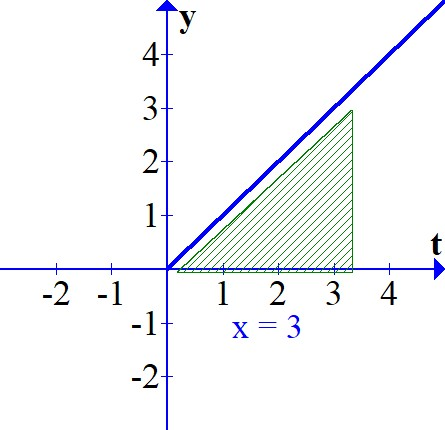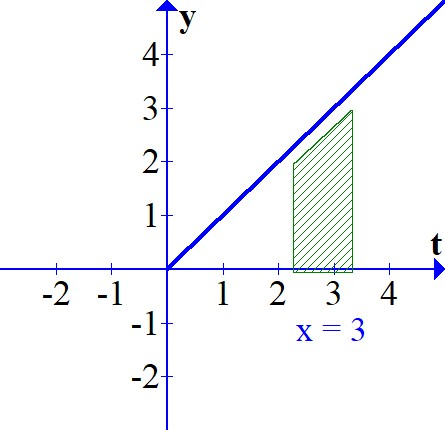# Let f(t) = t and consider the two area functions A(x) = \int_0^x f(t) dt and F(x) = \int_2^x f(t)...

## Question:

Let {eq}f(t) = t {/eq} and consider the two area functions {eq}A(x) = \int_0^x f(t) dt {/eq} and {eq}F(x) = \int_2^x f(t) dt. {/eq}

a. Evaluate {eq}A(2) {/eq} and {eq}A(4). {/eq} Then use geometry to find an expression for {eq}A(x), {/eq} for {eq}x \geq 0. {/eq}

b. Evaluate {eq}F(4) {/eq} and {eq}F(6). {/eq} Then use geometry to find an expression for {eq}F(x), {/eq} for {eq}x \geq 2. {/eq}

c. Show that {eq}A(x) - F(x) {/eq} is a constant and that {eq}A'(x) = F'(x) = f(x). {/eq}

## Second Fundamental Theorem of Calculus:

The Second Fundamental Theorem of Calculus states that, given a continuous function {eq}f(t) {/eq} defined on the interval {eq}a \leq t \leq b, {/eq} if {eq}a \leq x \leq b {/eq} and {eq}F(x) = \displaystyle\int_a^x f(t) \: dt, {/eq} then {eq}F'(x) = f(x). {/eq} The way this is proven is to revert back to the definition of the derivative:

{eq}F'(x) = \displaystyle\lim_{\Delta x \to 0} \frac{F(x + \Delta x) - F(x)}{\Delta x} {/eq}

and apply the Mean Value Theorem.

a. We are given the function {eq}f(t) = t {/eq} and the two area functions {eq}A(x) = \displaystyle\int_0^x f(t) \: dt {/eq} and {eq}F(x) = \displaystyle\int_2^x f(t) \: dt. {/eq} Therefore

{eq}\begin{eqnarray*}A(2) & =& \int_0^2 t \: dt \\ \\ & =& \displaystyle\frac{t^2}2 \biggr|_0^2 \\ \\ & =& 2 \end{eqnarray*} {/eq}

and

{eq}\begin{eqnarray*}A(4) & = & \int_0^4 t \: dt \\ \\ & =& \displaystyle\frac{t^2}2 \biggr|_0^4 \\ \\ & = & 8 \end{eqnarray*} {/eq}

The following graph displays this integral for {eq}x = 3: {/eq}This represents the area of a half a square with side length {eq}x, {/eq} so the answer is {eq}\displaystyle\frac{x^2}2. {/eq}

b. We have

{eq}\begin{eqnarray*}F(4) & = & \int_2^4 t \: dt \\ \\ & =& \displaystyle\frac{t^2}2 \biggr|_2^4 \\ \\ & =& 6 \end{eqnarray*} {/eq}

and

{eq}\begin{eqnarray*}F(6) & = & \int_2^6 t \: dt \\ \\ & = & \displaystyle\frac{t^2}2 \biggr|_2^6 \\ \\ & = & 16 \end{eqnarray*} {/eq}

The following graph displays this integral for {eq}x = 3: {/eq}This is a trapezoid, with height {eq}h = x - 2 {/eq} and bases {eq}b_1 = 2 {/eq} and {eq}b_2 = x. {/eq} Therefore, the area is

{eq}A = \displaystyle\frac{h(b_1 + b_2)}{2} = \displaystyle\frac{x^2}2 - 2. {/eq}

c. We have {eq}A(x) - F(x) = \displaystyle\frac{x^2}2 - \left( \displaystyle\frac{x^2}2 - 2 \right) = 2. {/eq} Furthermore, {eq}A'(x) = F'(x) = x = f(x). {/eq}